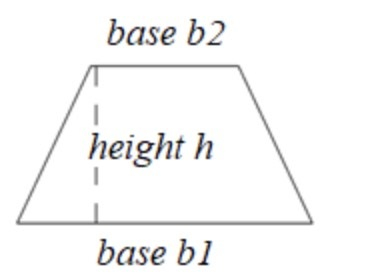# How To Find The Area of a Trapezium in Golang?

In this tutorial, we are going to see the Golang program to find the Area of a Trapezium. The area is the total space covered by any closed figure.## Formula

Area of Trapezium - ½ * (b1 + b2) * h
b1 - length of one parallel side of a Trapezium
b2 - length of another parallel side of a Trapezium
h - the distance between the parallel sides of a Trapezium.


For example, the length of one parallel side of a Trapezium is 10 cm and the other parallel side is 8 cm, and the distance between them is 4 so the Area of a Trapezium is −

b1 = 10 cm
b2 = 8 cm
h = 4 cm
Area = ½ * (a + b) * h
= ½ *( 10 + 8) * 4
= ½ * 18 * 4
= 9 * 4
= 32 cm^2


## Finding the Area of a Trapezium within the function

### Algorithm

Step 1 − Declaring the variables for the length of the parallel sides, the distance between the parallel sides, and the area of the float64 data type.

Step 2 − Initialize the variable.

Step 3 − Find the Area using the above formula within the function.

Step 4 − Printing the result.

Time Complexity:
O(1)

Space Complexity:
O(1)


## Example 1

In this example, we are going to find the Area of a Trapezium within the function.

package main
// fmt package provides the function to print anything
import (
"fmt"
)
func main() {
// declaring the floating variables using the var keyword for
// storing the length of the parallel sides of the Trapezium,
// distance between the parallel sides and also a variable area
// to store Area
var a, b, d, Area float64
fmt.Println("Program to find the Area of a Trapezium.")

// initializing the length of one of the parallel side of a Trapezium
a = 10

// initializing the length of another parallel side of a Trapezium
b = 8

// initializing the length of distance between parallel sides of a Trapezium
d = 4

// finding the Area of a Trapezium
Area = (1.0 / 2) * ((a + b) * d)

// printing the result
fmt.Println("The Area of a Trapezium whose length of the parallel sides and distance between the sides are", a,",", b,",", d, "is", Area, "cm * cm.")
fmt.Println("(Finding the Area of a Trapezium within the function)")
}


## Output

% go run tutorialpoint.go
Program to find the Area of a Trapezium.
The Area of a Trapezium whose length of the parallel sides and distance between the sides are 10 8 4 is 36 cm * cm.
(Finding the Area of a Trapezium within the function)


## Finding the Area of a Trapezium in the separate function

### Algorithm

Step 1 − Declaring the variables for the length of the parallel sides, the distance between the parallel sides, and the area of the float64 data type.

Step 2 − Initialize the variable.

Step 3 − Calling the function with the length of parallel sides of the trapezium and the as a parameter, and storing the Area the function is returning.

Step 4 − Printing the result.

## Example 2

In this example, we are going to find the Area of a Trapezium by defining the separate function to find the Area.

package main

// fmt package provides the function to print anything
import (
"fmt"
)

// in this line we have declared the function that have float64
// type parameter and float64 type returntype
func areaOfTrapezium(a, b, d float64) float64 {
// returning the area by applying the formula
return (1.0 / 2) * ((a + b) * d)
}
func main() {
// declaring the floating variables using the var keyword for
// storing the length of the parallel sides of the Trapezium,
// distance between the parallel sides and also a variable area
// to store Area
var a, b, d, Area float64
fmt.Println("Program to find the Area of a Trapezium.")

// initializing the length of one of the parallel side of a Trapezium
a = 10

// initializing the length of another parallel side of a Trapezium
b = 8

// initializing the length of distance between parallel sides of a Trapezium
d = 4

// finding the Area of a Trapezium by calling the function with the respective parameter
Area = areaOfTrapezium(a, b, d)

// printing the result
fmt.Println("The Area of a Trapezium whose length of the parallel sides and distance between the sides are", a,",", b,",", d, "is", Area, "cm * cm.")
fmt.Println("(Finding the Area of a Trapezium in the separate function)")
}


## Output

Program to find the Area of a Trapezium.
The Area of a Trapezium whose length of the parallel sides and distance between the sides are 10 , 8 , 4 is 36 cm * cm.
(Finding the Area of a Trapezium in the separate function)


## Conclusion

These are the two ways to find the Area of a Trapezium in Golang. The second way is much better in terms of modularity and code reusability as we can call that function anywhere in the project. To learn more about go you can explore these tutorials.